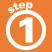Home    |    Teacher    |    Parents    |    Glossary    |    About UsIn the problem 3 x 4 = 12, 3 and 4 are factors and 12 is the product.

Factoring is like taking a number apart. It means to express a number as the product of its factors. Factors are either composite numbers or prime numbers (except that 0 and 1 are neither prime nor composite).

The number 12 is a multiple of 3, because it can be divided evenly by 3.

3 x 4 = 12
3 and 4 are both factors of 12
12 is a multiple of both 3 and 4.

Click each number below to see factors and multiples.

SHOW FACTORS                         SHOW MULTIPLES

 Homework Help | Pre-Algebra | FactoringEmail this page to a friendSearch·  Factors and     multiples·  Greatest     Common Factor (GCF)·  Least Common     Multiple (LCM)First Glance In Depth Examples WorkoutFactors and multiples